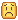# My character moves faster in diagonal, how to solve it?

I created a flying sistem for roblox, it is working fine and smoothly, the only problem is walkgin faster indiagonal sinse I’m using BodyVelocity and 1-based inputs (multiplied by walkspeed) to define its velocity.

the final code, simplified, is like:

``````-- W A S D Space and Alt Keys gives valor 1 or -1 to x, y or z.
vel.Velocity = Camera.CFrame:VectorToWorldSpace(Vector3.new(x,y,z)) * Humanoid.WalkSpeed
``````

But i just need to know what to do with this to simplify:

``````Vector3.new(x,y,z) * Humanoid.WalkSpeed
``````

A BodyGyro is keeping it facing to the CameraCFrame.LookVector.

This makes the sum of vector * Humanoid.WalksPeed get a final velocity higher then Walkspeed in diagonal.

HOW WOULD I OVERCOME THIS???

Here below is a graph of how the movement is working

This video shows the problem, you can see by the output that Magnitude is higher when moving in global diagonals (also notable by eye on char):

3 Likes

Just .Unit it as we only care about the direction of the vector and not the magnitude caused by the pythagoras triangle thingy from the vector summation.

``````Camera.CFrame:VectorToWorldSpace(Vector3.new(x,y,z)).Unit
``````

But then you also have to make sure you don’t .Unit (0,0,0) so we put a NanCheck for that

``````local function isnan(arg: any): boolean
return not rawequal(arg, arg)
end

local movementDirection = Camera.CFrame:VectorToWorldSpace(Vector3.new(x,y,z)).Unit
if isnan(movementDirection.X) then
vel.Velocity = Vector3.new(0,0,0)
else
vel.Velocity = movementDirection * Humanoid.WalkSpeed
end
``````

Edit 2: Try this out @Necro_las

4 Likes

Oof, i thought it had worked but noLooks like the function is not returning true when (NAN,NAN,NAN)

and using unit beaks the ability of stopping in air, keeps me in constant movement.

1 Like
• It does stop in the air now and the isnan() function is fine.
But meeh ill have to work around for the acceleration.

Since you are working with Forces I recommend using PID instead to control the acceleration better to achieve a certain velocity.

Edit: Here is another method to measure movement direction and to do a NAN check

``````	if walkDir.Magnitude > 0 then --(0, 0, 0).Unit = NaN, do not want
walkDir = walkDir.Unit --Normalize, because we (probably) changed an Axis so it's no longer a unit vector
end
``````

Change this

`Vector3.new(x,y,z) * Humanoid.WalkSpeed`

to this:

`Vector3.new(x,y,z) / Vector3.new(x,y,z).Magnitude * Humanoid.WalkSpeed`

This will convert the vector value into a unit vector.

This is the solution: multiply Vector.Unit by the clamped magnitude of the vector. This will not cut acceleration by using Unit. The only tiny detail left is that this will not avoid the acceleration in diagonal from beeing faster, but velocity will be clamped to your max velocity.

``````local function isnan(arg: any): boolean
return not rawequal(arg, arg)
end
``````
``````local Vector = Camera.CFrame:VectorToWorldSpace(Vector3.new(x,y,z))
local Unit = Vector.Unit

if isnan(Unit.X) then
Unit = Vector3.new(0,0,0)
end

vel.Velocity = Unit * (math.clamp(Vector.Magnitude, 0, 1)) * FlySpeed.Value
``````

@dthecoolest thanks for the help, ure a genius <3
got tips from here: [Unity C#] First Person Controller (E02: Fixing Diagonal Movement Speed) - YouTube

3 Likes